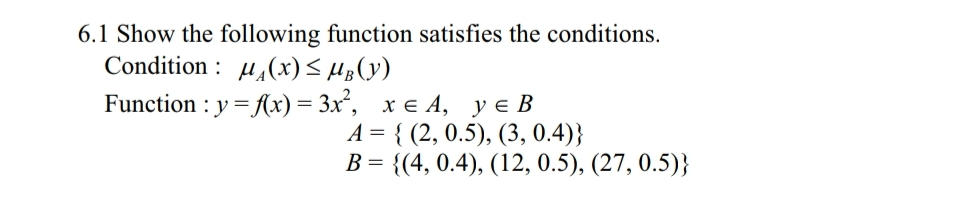# 6.1 Show the following function satisfies the conditionsCondition:Function : y-(x)-3x,y є Bx є 4,A-{ (2, 0.5), (3, 0.4))B 4, 0.4), (12, 0.5), (27, 0.5))

Question
40 views

the book is "First Course on Fuzzy Theory and Applications"help_outlineImage Transcriptionclose6.1 Show the following function satisfies the conditions Condition: Function : y-(x)-3x, y є B x є 4, A-{ (2, 0.5), (3, 0.4)) B 4, 0.4), (12, 0.5), (27, 0.5)) fullscreen
check_circle

Step 1

To show: The given function satisfies the condition

Step 2

Given: The function is

Step 3

From the gi...

### Want to see the full answer?

See Solution

#### Want to see this answer and more?

Solutions are written by subject experts who are available 24/7. Questions are typically answered within 1 hour.*

See Solution
*Response times may vary by subject and question.
Tagged in

### Math